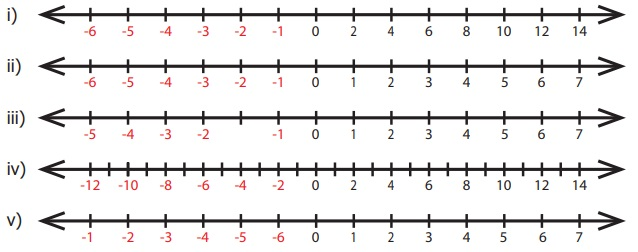Home | | Maths 6th Std | Exercise 2.1

# Exercise 2.1

6th Maths : Term 3 Unit 2 : Integers : Exercise 2.1 : Fill in the blanks, Say True or False, Objective Type Questions, Text Book Back Exercises Questions with Answers, Solution

Exercise 2.1

Fill in the blanks

i) The potable water available at 100m below the ground level is denoted as −100  m.

ii) A swimmer dives to a depth of 7 feet from the ground into the swimming pool. The integer that represents this, is −7 feet.

iii) −46 is to the left of −35 on the number line.

iv) There are 11 integers from −5 to +5 (both inclusive).

v) 0 is an integer which is neither positive nor negative.

2. Say True or False

i) Each of the integers −18, 6, −12, 0 is greater than −20. [True]

ii) −1 is to the right of 0. [ False]

iii) −10 and 10 are at equal distance from 1. [ False]

iv) All negative integers are greater than zero. [ False]

v) All whole numbers are integers. [True]

3. Mark the numbers 4, −3, 6, −1 and −5 on the number line.4. On the number line, which number is

i) 4 units to the right of −7?

ii) 5 units to the left of 3?

5. Find the opposite of the following numbers.

i) 44

ii) −19

iii) 0

iv) −312

v) 789

i) 44 = Opposite of 44 is −44

ii) −19 = Opposite of −19 is +19

iii) 0 = It has no sign

iv) − 312 = Opposite of −312 is + 312

v) 789 = Opposite of 789 is −789

6. If 15 km east of a place is denoted as + 15 km, what is the integer that represents 15 km west of it?

7. From the following number lines, identify the correct and the wrong representations with reason.i) Wrong Integers are not continuously marked.

ii) Correct Integers are correctly marked.

iii) Wrong Integer − 2 is marked wrongly.

iv) Correct Integers are marked at equal distance.

v) Wrong negative integers marked wrongly.

8. Write all the integers between the given numbers.

i) 7 and 10

ii) −5 and 4

iii) −3 and 3

iv) −5 and 0

i) 7 and 10 = 8, 9

ii) −5 and 4 = −4, −3, −2, −1, 0, 1, 2, 3

iii) −3 and 3 = −2, −1, 0, 1, 2

iv) −5 and 0 = −4, −3, −2, −1

9. Put the appropriate signs as <, > or = in the box.

i) −78

ii) −8−7

iii) −999−1000

iv) −111−111

v) 0−200

i) −7 < 8

ii) −8 <  −7

iii) −999 > −1000

iv) −111 = − 111

v) 0 > − 200

10. Arrange the following integers in ascending order.

i) −11, 12, −13, 14, −15, 16, −17, 18, −19, −20

−20, −19, −17, −15, −13, −11, 12, 14, 16, 18

ii) −28, 6, −5, −40, 8, 0, 12, −1, 4, 22

−40, −28, −5, −1, 0, 4, 6, 8, 12, 22

iii) −100, 10, −1000, 100, 0, −1, 1000, 1, −10

−1000, −100, −10, −1, 0, 1, 10, 100, 1000

11. Arrange the following integers in descending order.

i) 14, 27, 15, −14, −9, 0, 11, −17

27, 15, 14, 11, 0, −9, −14, −17

ii) −99, −120, 65, −46, 78, 400, −600

400, 78, 65, −46, −99, −120, −600

iii) 111, −222, 333, −444, 555, −666, 7777, −888

777, 555, 333, 111, −222, −444, −666, −888

Objective Type Questions

12. There are __________ positive integers from −5 to 6.

a) 5

b) 6

c) 7

d) 11

13. The opposite of 20 units to the left of 0 is

a) 20

b) 0

c) −20

d) 40

14. One unit to the right of −7 is...........

a) +1

b) −8

c) −7

d) −6

15. 3 units to the left of 1 is

a) −4

b) –3

c) −2

d) 3

16. The number which determines marking the position of any number to its opposite on a number line is

a) −1

b) 0

c) 1

d) 10

Exercise 2.1

1. i) –100 ii) −7 iii) left iv) 11 v) 0

2. i) True ii) False iii) False iv) False v) True

3.4. i) −3 ii) −2

5. i) −44 ii) +19 or 19 iii) 0 iv) 312 v) −789

6. i) −15 km

7. i) Wrong, Integers are not continuously marked

ii) Correct, Integers are correctly marked.

iii) Wrong, Integer −2 is marked wrongly.

iv) Correct, Integers are marked at equal distance.

v) Wrong, negative integers marked wrongly.

8. i) 8, 9 ii) −4, −3, −2, −1, 0, 1, 2, 3 iii) −2, −1, 0, 1, 2 iv) −4, −3, −2, −1

9. i) −7 < 8 ii) −8 < −7 iii) −999 >−1000 iv) −111 = −111 v) 0 > −200

10. i) −20, −19,−17, −15, −13, −11, 12, 14, 16, 18

ii) −40, −28, −5, −1, 0, 4, 6, 8, 12, 22

iii) −1000, −100, −10, −1,0, 1, 10, 100, 1000

11. i) 27, 15, 14, 11, 0, −9, −14, −17

ii) 400, 78, 65, −46, −99, −120, −600

iii) 777, 555, 333, 111, −222, −444, −666, −888

12. c) 7

13) a) 20

14) d) −6

15) c) −2

16) b) 0

Tags : Questions with Answers, Solution | Integers | Term 3 Chapter 2 | 6th Maths , 6th Maths : Term 3 Unit 2 : Integers
Study Material, Lecturing Notes, Assignment, Reference, Wiki description explanation, brief detail
6th Maths : Term 3 Unit 2 : Integers : Exercise 2.1 | Questions with Answers, Solution | Integers | Term 3 Chapter 2 | 6th Maths